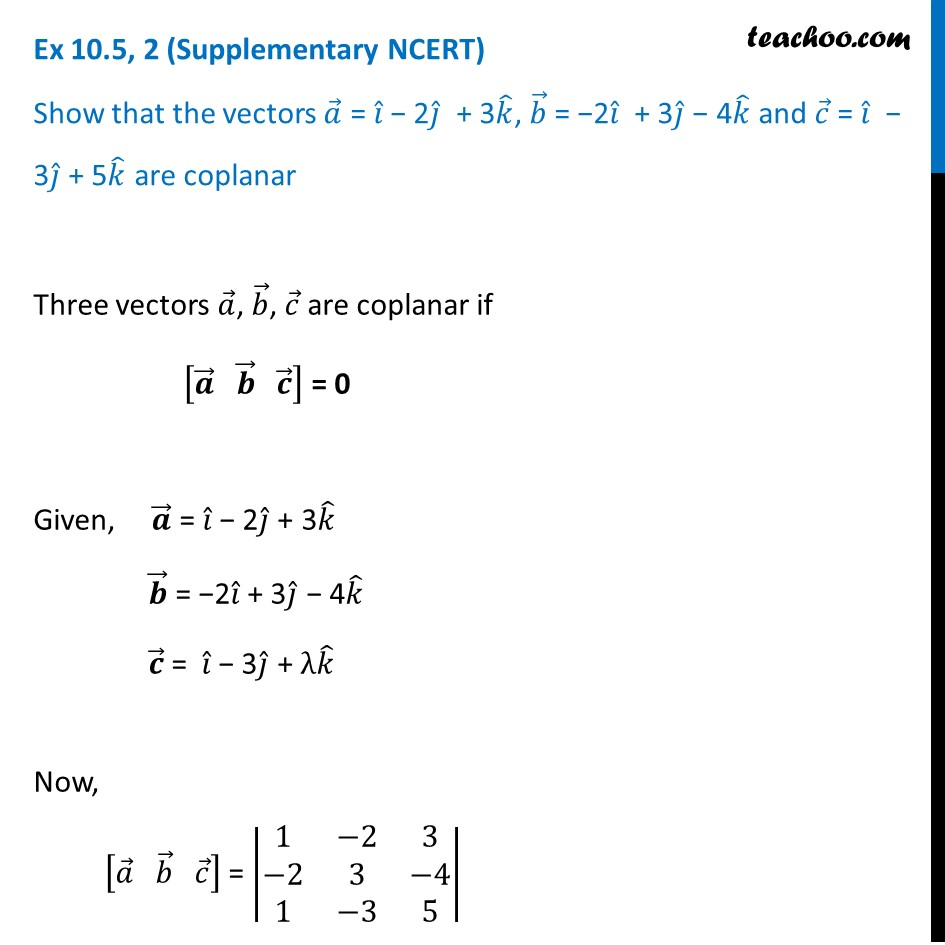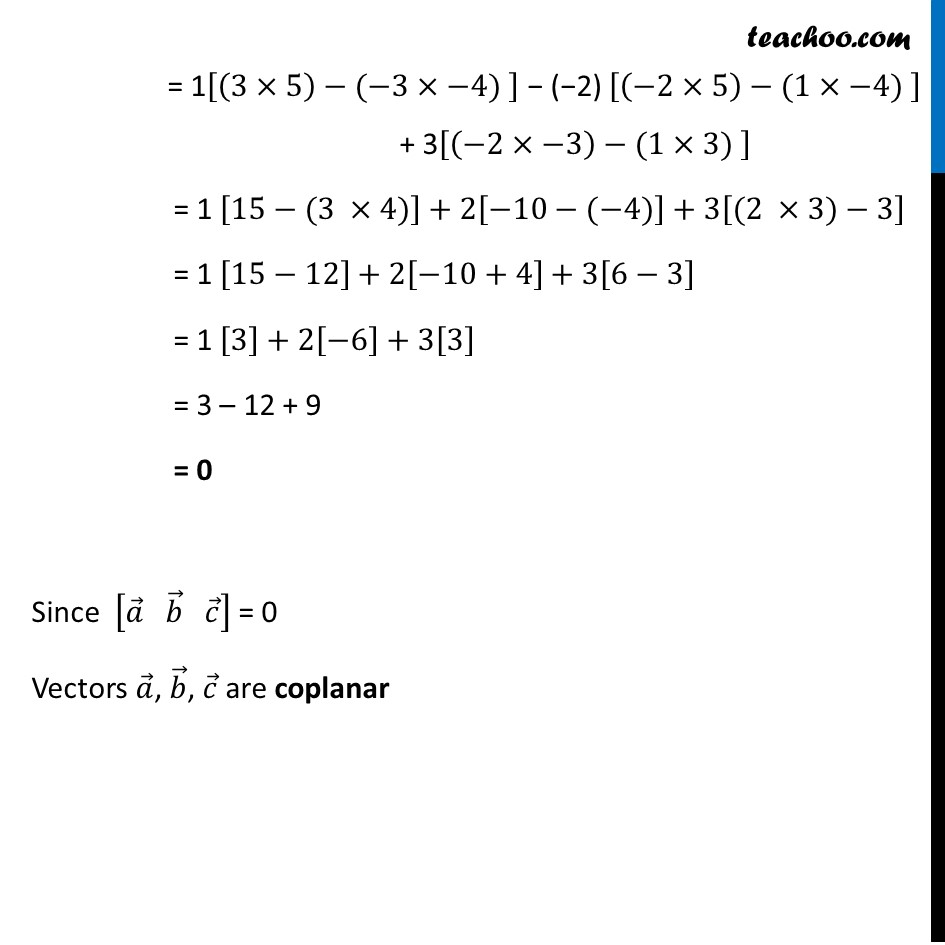Ex 10.5 (Supplementary NCERT)

Chapter 10 Class 12 Vector Algebra
Serial order wise

This question is the same as Example 27 (Supplementary NCERT)Learn in your speed, with individual attention - Teachoo Maths 1-on-1 Class

### Transcript

Ex 10.5, 2 (Supplementary NCERT) Show that the vectors 𝑎 ⃗ = 𝑖 ̂ − 2𝑗 ̂ + 3𝑘 ̂, 𝑏 ⃗ = −2𝑖 ̂ + 3𝑗 ̂ − 4𝑘 ̂ and 𝑐 ⃗ = 𝑖 ̂ − 3𝑗 ̂ + 5𝑘 ̂ are coplanarThree vectors 𝑎 ⃗, 𝑏 ⃗, 𝑐 ⃗ are coplanar if [𝒂 ⃗" " 𝒃 ⃗" " 𝒄 ⃗ ] = 0 Given, 𝒂 ⃗ = 𝑖 ̂ − 2𝑗 ̂ + 3𝑘 ̂ 𝒃 ⃗ = −2𝑖 ̂ + 3𝑗 ̂ − 4𝑘 ̂ 𝒄 ⃗ = 𝑖 ̂ − 3𝑗 ̂ + λ𝑘 ̂ Now, [𝑎 ⃗" " 𝑏 ⃗" " 𝑐 ⃗ ] = |■8(1&−2&3@−2&3&−4@1&−3&5)| = 1[(3×5)−(−3×−4) ] − (−2) [(−2×5)−(1×−4) ] + 3[(−2×−3)−(1×3) ] = 1 [15−(3 ×4)]+2[−10−(−4)]+3[(2 ×3)−3] = 1 [15−12]+2[−10+4]+3[6−3] = 1 +2[−6]+3 = 3 – 12 + 9 = 0 Since [𝑎 ⃗" " 𝑏 ⃗" " 𝑐 ⃗ ] = 0 Vectors 𝑎 ⃗, 𝑏 ⃗, 𝑐 ⃗ are coplanar